# Linear Algebra for Data Science: Why?

• Part 0: Why using linear algebra for Data Science?
• Part 1: The three definitions of a vector
• Part 2: Linear combinations, span, and basis
• Part 3: Linear transformations and matrices
• Part 4: Matrix multiplication
• Part 5: Determinant
• Part 6: Images, kernel, and inverses
• Part 7: Rectangular matrices
• Part 8: Special matrices
• Part 9: Scalar product and duality
• Part 10: Vectorial product
• Part 11: Change of basis
• Part 12: Eigenvectors and eigenvalues
• Part 13: Singular-value decomposition (SVD)
• Part 14: Norms
• Part 15: Moore-Penrose pseudo inverse
• Part 16: Trace
• Part 17: Principal Component Analysis

Whenever I create courses for data science, I get asked the same question over and over:

What math do I need to know?

And my reply is usually a bit vague: It depends.

And really, it does!

Reality is that you can do data science with just some highschool-level knowledge of math.

I have even worked with guys who created a Deep Learning course with almost no math prerequisite.

The idea is to focus only on developing the intuition, and then practice. Practice, practice, practice.

So… no math.

But what if you want to go further? What if you want a long and successful career as a data scientist?

Then you have no choice, you will have to know the math.

Note: This is an introductory article and I will mention a few linear algebra concepts. If you know nothing about it, don’t worry. We’ll get back to it in future articles. All you need to know is some highschool math.

## Why should you care about Linear Algebra?

A few years ago, when I first encountered the field, I hated it.

It felt like a bunch of abstract and useless theorems, I had no idea why I was learning that, and, more importantly, I had absolutely no intuition about all of it.

I was helpless.

Until recently..

When I decided it may be a good idea to understand what I was going.

So.. why?

All machine learning algorithms DO use Linear Algebra.

All of them.

And that’s also true for statistical models.

Take regression.

The simplest ever model.

All you have to do is draw a line in the middle of a scatterplot.

People did that way before the Legendre started to develop the theory of regression!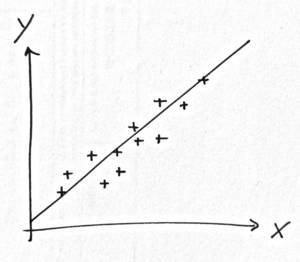How would you draw this line?

Intuitively, it’s easy. You just do it.

But.. mathematically?

What we do is minimize the vertical distances between each point and the line.

So, if you have a line $y = \beta_0 x + \beta_1$, what we want is to estimate $\beta_0$ and $\beta_1$.

Mathematically, you the sum of distances:

and then minimize it. To do so, compute the partial derivatives, solve the equation of these derivatives equal to zero, and.. that’s it! You’re done.

So..

..where are the stats?

.

No stats.

No mean, no standard deviation, no statistical distribution, no confidence interval.. no stats.

Just solving an equation.

And I can even make predictions with this line!

So.. why do people say you need statistics for a regression analysis?

In fact, you will need statistics only if you want to dig deeper.

If you want a confidence interval, for instance.

Or if you want to know the properties of your estimator. The bias, the convergence, the variance. That kind of things.

And THAT is important too.

But..

To minimize the sum above?

No stats.

All I need really…

.

.

I need linear algebra!

Exactly!

Solving the equation was actually linear algebra.

Even if it didn’t look that way, we projected a 2-dimension space into a 1-dimension subspace and then computed a Jacobian.

We didn’t notice because it was a simple example.

But if we tried to solve a multiple regression with $p$ variables, it’d be more obvious.

All machine learning algorithms and all statistical models use linear algebra.

All of them without exception.

## So… what’s the point?

Who knows PCA here?Who knows PCA theory and can explain it to me?Yea..

I thought so.

But really, if you raised your hand for the first question and not the second, there is no shame to have.

In fact, I was in the same position not so long ago.

(And if you haven’t raised your hand at all, it’s okay too! You’re here to learn.)

It’s pure logic.

How to do PCA?

One line of code.

How to explain PCA?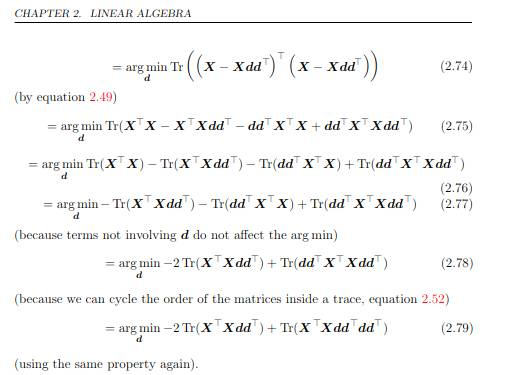Screenshot from the book Deep Learning from I. Goodfellow, Y. Bengio, and A. Courville, chapter 2 Linear Algebra on PCA.

There are three pages like that.

So.. naturally, that looks less cool.

And when the client asks to do a PCA, are we really going to suck it up for hours while the results will look exactly the same?

Well.. yes.

There are two reasons for it.

First, I don’t know about you, but I’m kind of embarassed to say I’m a data scientist but I’m unable to explain (or even understand) the algorithms I use on a daily basis..

Reminds me of this: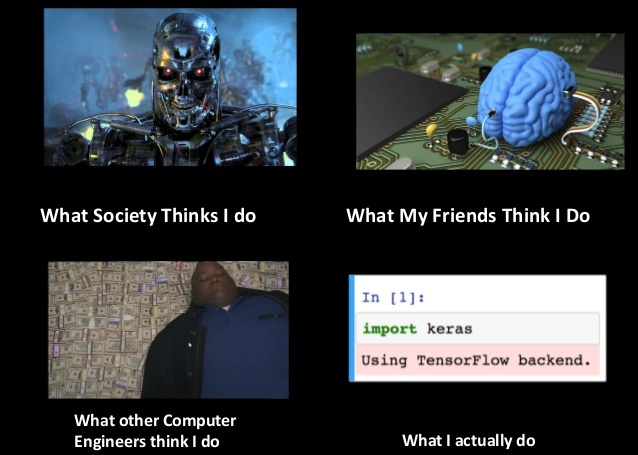Image that appeared all over the internet

Well, no.

It sucks to be the guy who’s just applying algorithms like a monkey.

I feel like a fraud.

Plus, my real job is actually more complicated than that.

Not fun.

Second reason is that when a client hires me for a data science gig, he expects more from me that that just applying an algorithm.

A client actually asked me recently to perform a PCA.

And.. I had to get results that were not directly from R outputs!

I felt stupid just because I had to do something more than push a button (yea.. don’t judge me).

But I took it as an opportunity.

I went back into PCA theory, learned how it works, why, what you do with it, etc.

And that was really cool!

I had learned it at school, but, you know.. we forget everything.

So not only was it cool to learn it again, but more importantly I could make my client satisfied!

And PCA, by the way, is purely linear algebra.

We’ll get back on this topic in a few articles :)

## But what IS linear algebra exactly?

I used to ignore it.

When I was at school, the term just appeared out of the blue, and nothing explained it to me.

What does linear mean exactly?

Well, I quickly understood that some functions were not linear.

Sinus, cosinus, square root, exponential, logarithm… All of them: not linear.

I also quickly got that doing additions was linear.

But what about a clear and rigorous definition?

It exists.

We learned it, and I found it back on Wikipedia: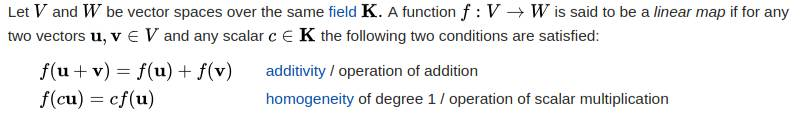..

Meh.

Not really intuitive, right?

My objectif in this article series is to go above this kind of definitions.

I prefer definitions that may be less rigorous.. but more intuitive.

.

A function is linear if it is written this way: $f(x) = ax$.

And the term linear comes from line.

You probably noticed.. we just wrote the equation of a line, one that passes through the origin.

It works with multiple variables too: $f(x, y) = ax + by$.

That’s the equation of a plan (that passes through the origin).

Linear algebra is simply the study of these functions.

That’s all there is.

Now, usually, we manipulate functions that are in spaces of $m$ and $n$ dimensions.

That’s a bit more complex..

But if we keep dimensions low, especially in 2 dimensions, we can visualize them, and now it gets really cool!

Really!

See, a function (or a transformation) in 2 dimensions is linear if it verifies the following two properties:

1. All lines remain lines.
2. The origin stays fixed.

See by yourself with the following transformation:

For instance, this function will transform the point with coordinates $(1, 1)$ into a new point with coordinates $(3, 2)$.

If it takes all the points of a line, then the transformed points still are in line.

And since it keeps the origin $(0, 0)$ at the same point after transformation, we conclude it is a linear transformation.

Look at it: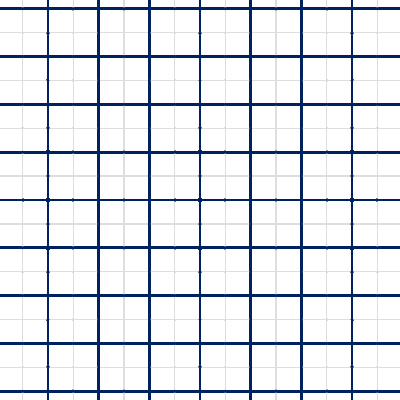Now, look at a transformation that is NOT linear: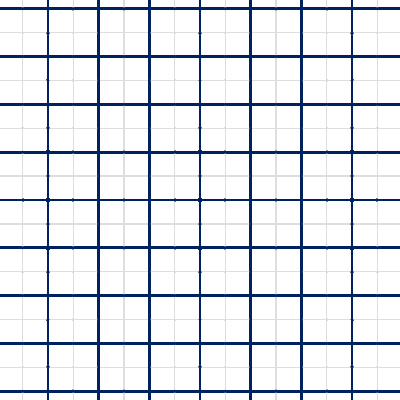First, the origin moves. It lands on the point with coordinates $(1, 0)$.

Second, lines clearly do not remain lines.

So.. not linear.

.

We’ll get back to these principles in future articles.

For now, your takeaway is: Linear algebra is the study of transformations where lines remain lines.

And just with that..

We can do a hell lot of things!

If that got you excited you want to know more… well.. just keep reading to the next article:

Part 1: The three definitions of a vector (soon)

Updated: